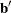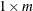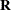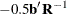Point Models

The expanded set of independent variables generated from the POINT, EPOINT, and QPOINT expansions can be used to perform ideal point regressions (Carroll, 1972) and compute ideal point coordinates for plotting in a biplot (Gabriel, 1981). The three types of ideal point coordinates can all be described as transformed coefficients. Assume that m independent variables are specified in one of the three point expansions. Letbe arow vector of coefficients for these variables and one of the dependent variables. Letbe a matrix created from the coefficients of the extra variables. When coordinates are requested with the MPC, MEC, or MQC o-option,andare created from multiple regression coefficients. When coordinates are requested with the CPC, CEC, or CQC o-option,andare created from canonical coefficients.

If you specify the POINT expansion in the MODEL statement,is anidentity matrix times the coefficient for the sums of squares (_ISSQ_) variable. If you specify the EPOINT expansion,is andiagonal matrix of coefficients from the squared variables. If you specify the QPOINT expansion,is ansymmetric matrix of coefficients from the squared variables on the diagonal and crossproduct variables off the diagonal. The MPC, MEC, MQC, CPC, CEC, and CQC ideal point coordinates are defined as. Whenis singular, the ideal point coordinates are infinitely far away and are set to missing, so you should try a simpler version of the model. The version that is simpler than the POINT model is the vector model, where no extra variables are created. In the vector model, designate all independent variables as IDENTITY. Then draw vectors from the origin to the COEFFICIENTS points.

Typically, when you request ideal point coordinates, the MODEL statement should consist of a single transformation for the dependent variables (usually IDENTITY, MONOTONE, or MSPLINE) and a single expansion for the independent variables (one of POINT, EPOINT, or QPOINT).